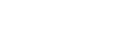## Top users rep per x weeks

3

(click on this box to dismiss)Q&A for Ubuntu users and developers

``````-- numofweeks: Number of weeks back in time
-- top: The top number to return

declare @numofweeks int
set @numofweeks=##numofweeks?2##

-- complete
select top ##top?10## dimtop.userid as [User Link]
-- debug
/* , dimtop.*
, se.suggestededit_rep
, bounty.[start]
, bounty.[close] */
, dimtop.estimatedrep
+ coalesce(se.suggestededit_rep,0)
- coalesce(bounty.[start],0)
+ coalesce(bounty.[close],0) as SumRepPeriod
from
(
-- aggregates per user (joined on post)
select usr.id as userid
, sum(upvote_count) as upvote_count
, sum(downvote_count) as downvote_count
, sum(upvote_score) as upvote_score
, sum(downvote_score) as downvote_score
, sum(Accepted_score) as Accepted_score
, sum(EstimatedRep) as EstimatedRep
from
(
-- aggregates per post
select postid
, sum(upv) as upvote_count
, sum(downv) as downvote_count
, sum(upvscore) as upvote_score
, sum(dvscore) as downvote_score
, sum(accrep) as Accepted_score
, sum(upvscore)
- sum(dvscore)
+ sum(accrep) as EstimatedRep
from (
select  p.id as postid
, case when p.posttypeid = 1 then 'Q' else 'A' end as QA
-- , min(p.owneruserid) as uid
, count(*) as upv
, 0 as downv
, case when p.posttypeid = 1 then count(*)*5  -- Q
when p.posttypeid = 2 then count(*)*10 -- A
end as upvscore
, 0 as dvscore
, 0 as accrep
from posts p
inner join votes v on v.postid = p.id
where v.votetypeid = 2 --UpVote
and v.creationdate between  Dateadd(ww, -@numofweeks, getdate()) and getdate()
group by p.posttypeid,  p.id
union
select p.id
, case when p.posttypeid = 1 then 'Q' else 'A' end
-- , min(p.owneruserid)
, 0
, count(*)
, 0
, case when p.posttypeid = 1 then count(*)*-2 -- Q
when p.posttypeid = 2 then count(*)*-2 -- A
end
, 0
from posts p
inner join votes v on v.postid = p.id
where v.votetypeid = 3 -- downvote
and v.creationdate between  Dateadd(ww, -@numofweeks, getdate()) and getdate()
group by p.posttypeid, p.id
union
select p.id
, 'A'
--, min(p.owneruserid)
, 0
, 0
, 0
, 0
, count(*)*15
from posts p
inner join votes v on v.postid = p.id
where v.votetypeid = 1
and p.posttypeid=2
and v.creationdate between  Dateadd(ww, -@numofweeks, getdate()) and getdate()
group by  p.id
) as fact
group by postid
) as dimension
inner join posts pst on dimension.postid = pst.id
group by usr.id
) as dimtop
left outer join
-- suggested edit for a user
(select count(*) * 2 as suggestededit_rep, owneruserid
from suggestededits
where approvaldate  between  Dateadd(ww, -@numofweeks, getdate())
and getdate()
group by owneruserid
) as se on se.owneruserid = dimtop.userid
left outer join
-- bounties for a user
(select   sum( case when votetypeid =8 then bountyamount else 0 end) as [start]
, sum( case when votetypeid =9 then bountyamount else 0 end) as [close]
, p.owneruserid
inner join posts p on p.id = v.postid
where votetypeid in (8,9)
and ((p.posttypeid = 2 and votetypeid =9)
or (p.posttypeid = 1 and votetypeid =8))
and v.creationdate between dateadd(ww,-@numofweeks,getdate()) and getdate()
group by p.owneruserid
) as bounty on bounty.owneruserid = dimtop.userid
order by dimtop.estimatedrep
+ coalesce(se.suggestededit_rep,0)
- coalesce(bounty.[start],0)
+ coalesce(bounty.[close],0) desc``````

### Enter Parameters

Options:
-Hold tight while we fetch your results
:records returned in :time ms:cached